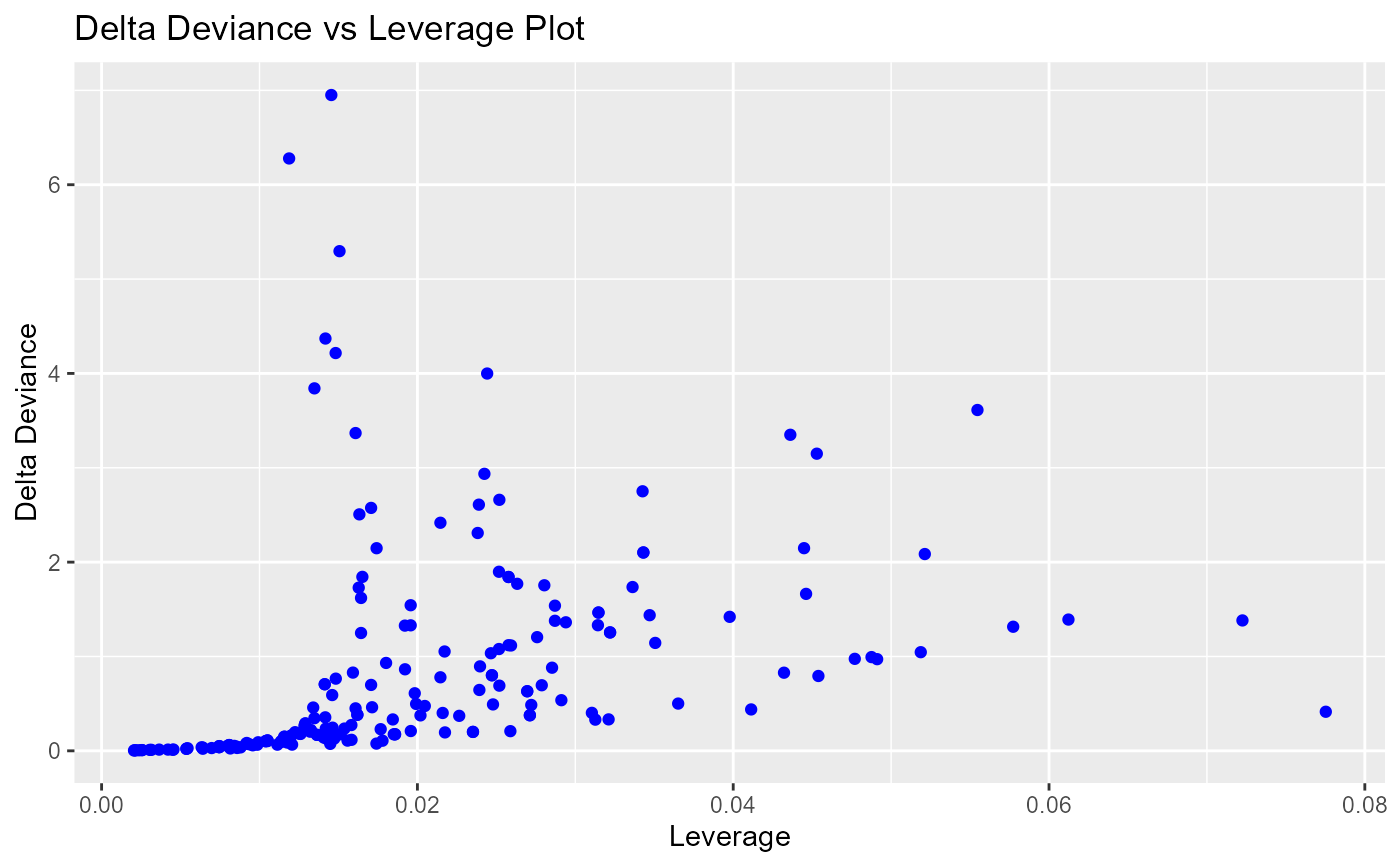Delta deviance vs leverage plot.

blr_plot_difdev_leverage(
model,
point_color = "blue",
title = "Delta Deviance vs Leverage Plot",
xaxis_title = "Leverage",
yaxis_title = "Delta Deviance"
)

## Arguments

model An object of class glm. Color of the points. Title of the plot. X axis label. Y axis label.

## Examples

model <- glm(honcomp ~ female + read + science, data = hsb2,